# OECD Material productivity data analysis 2 - Using R ggplot2 for making some graphs.Photo by Mateusz Klein on Unsplash

This post is following of the above post.
Let's make some graphs to get big picuture of the data.

Fisrstly, I make histograms for each variables.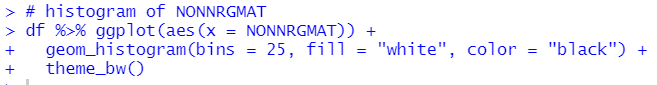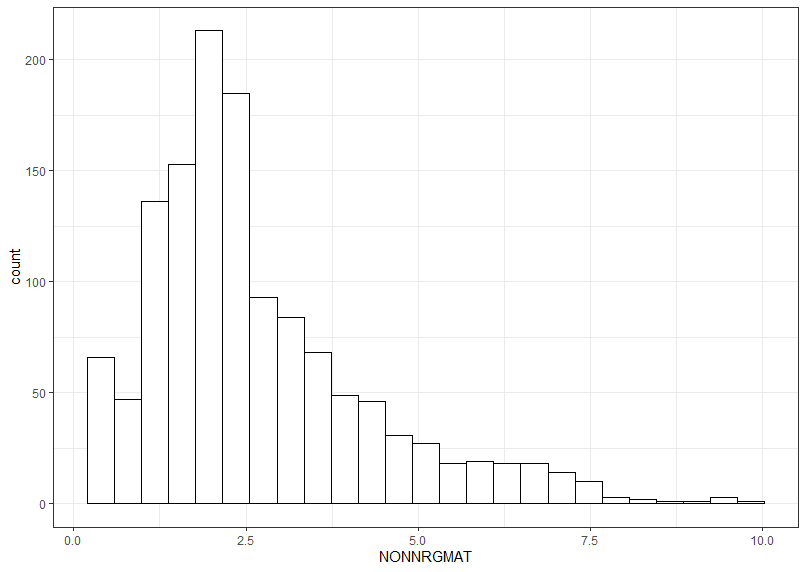TOTMAT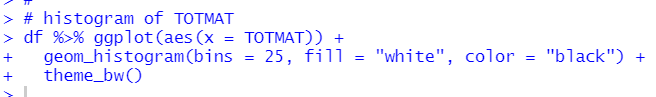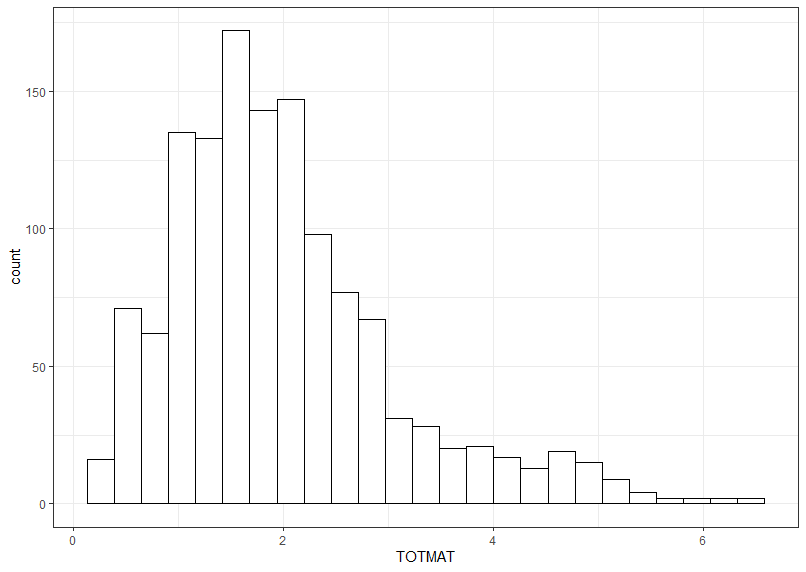before making gdp histogram, I load gridExtra package to arrange two histogram.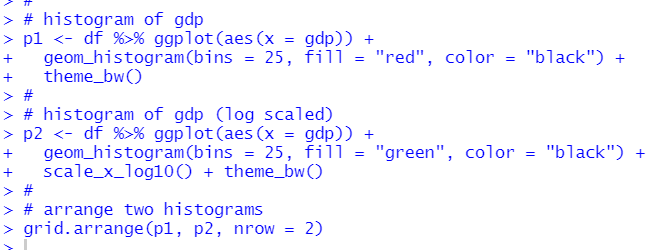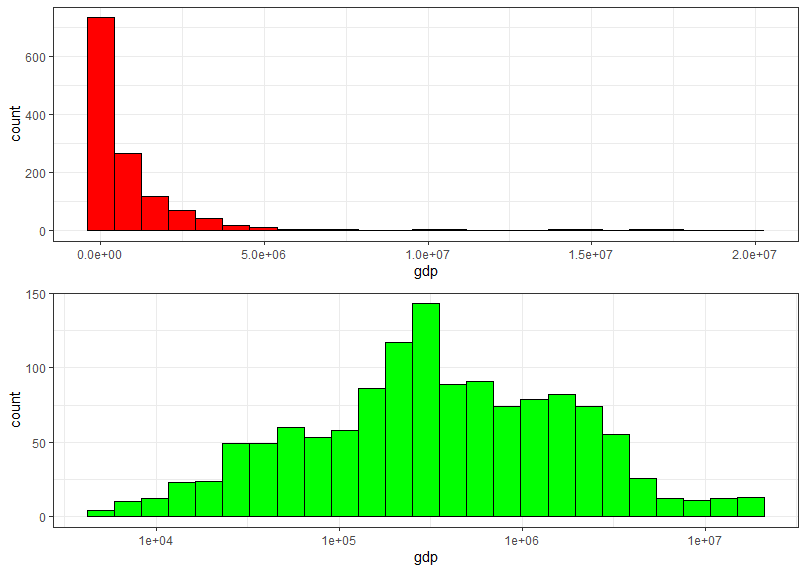The lower histogram is log-scaled histogram. So, it is betther to use log(gdp) for analysis later.

So, I make log(gdp) as l_gdp.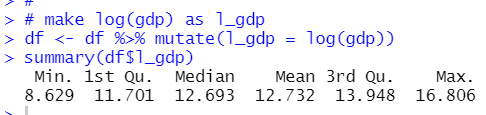capi histogram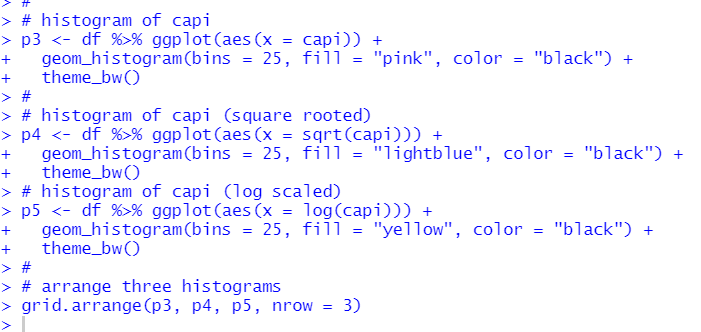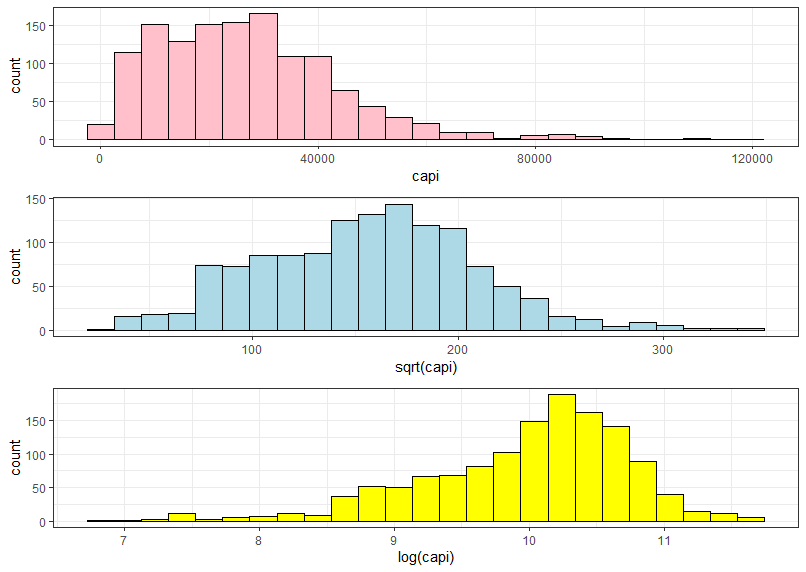I see square rooted capi is the most synmetric distribution. So I make sqare rooted capi as r_capi.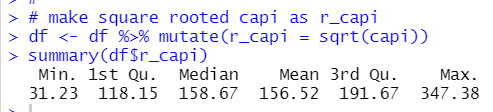Then, Let's see ranking by LOCATION for each variables.

Firstly, I check which TIME has the most observations.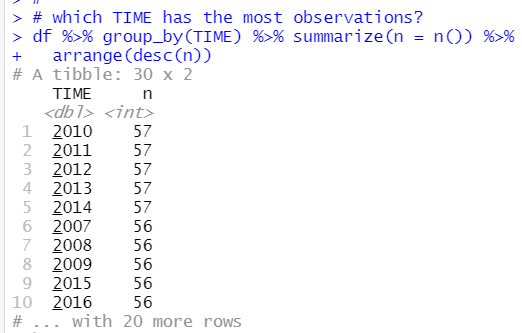2010, 2011, 2012, 2013 and 2014 has 57 observations.

So, I use 2010 ~ 2014 data only.

NONNRGMAT ranking by LOCATION.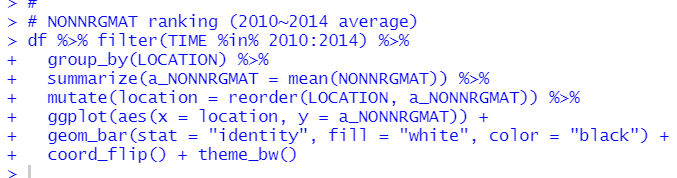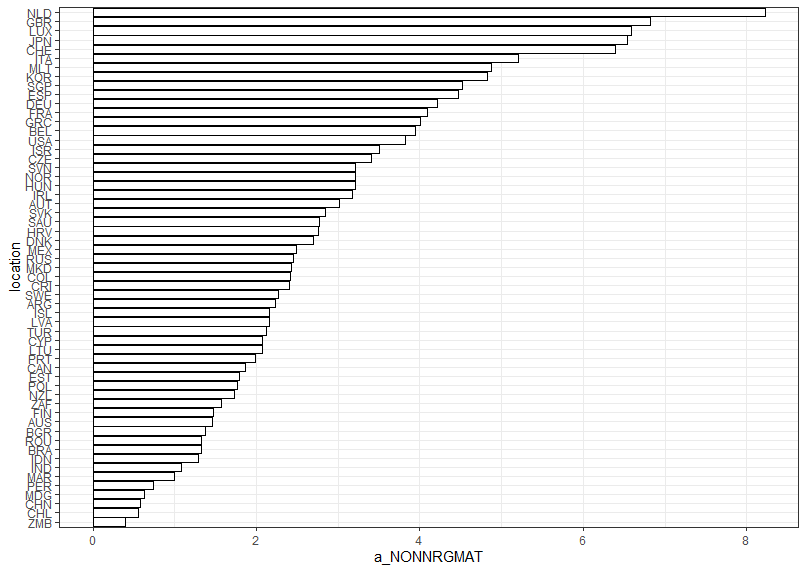NLD is the 1st, GBR is the 2nd, LUX is the 3rd.

TOTMAT ranking by LOCATION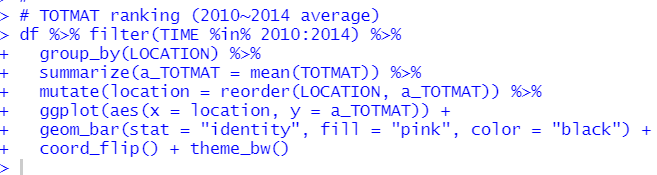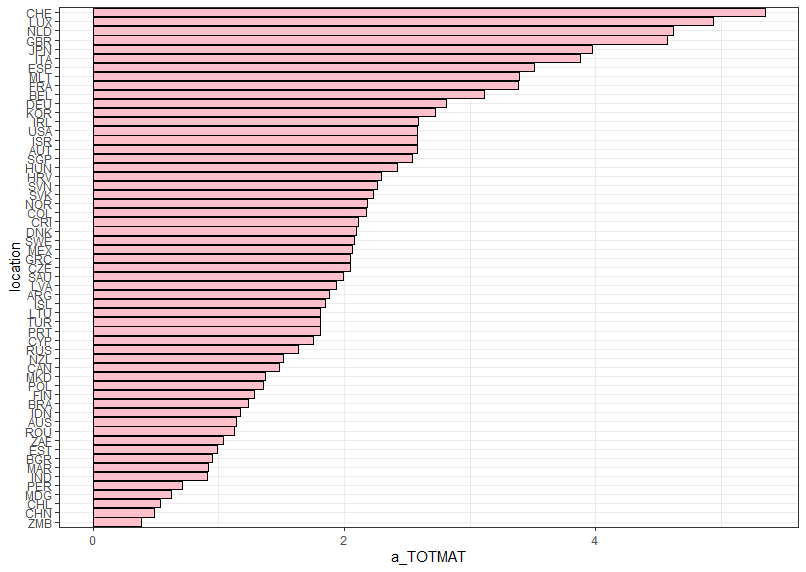CHE is the 1st,  LUX is the 2nd, NLD is the 3rd.

log(gdp) ranking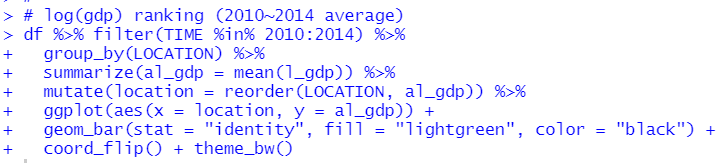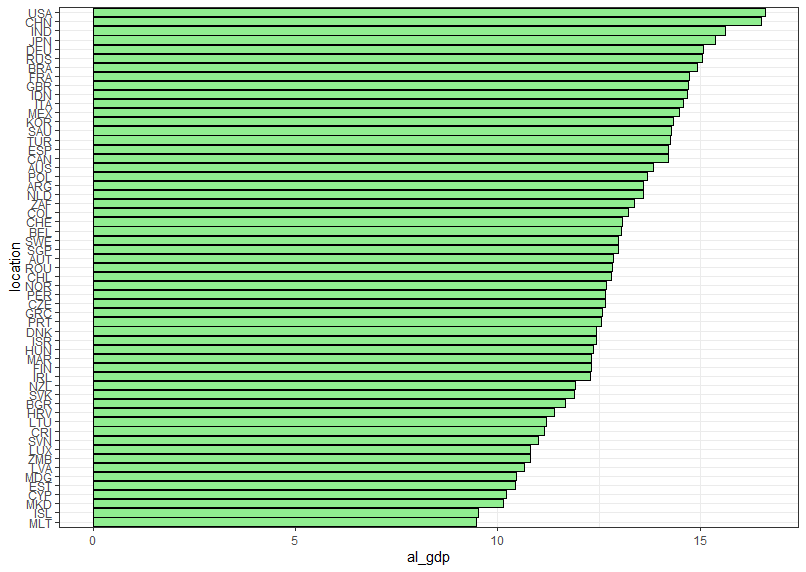USA is the 1st, CHN is the 2nd, IND is the 3rd.

sqrt(capi) ranking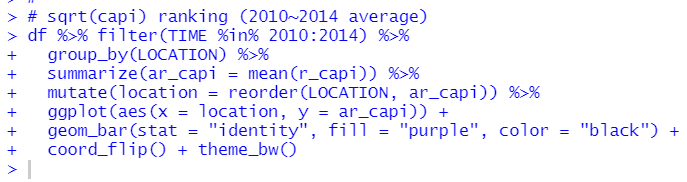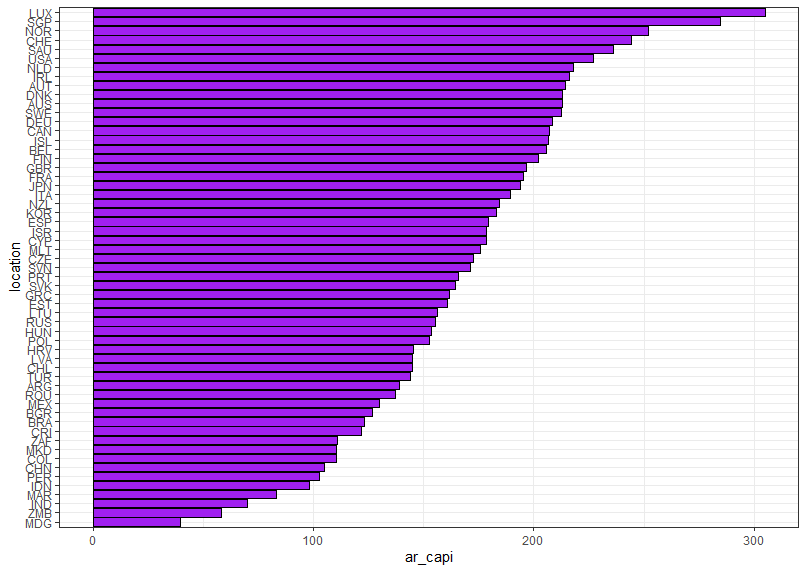LUX is the 1st, SGP is the 2nd, NOR is the 3rd.

Lastly, let's make scatter plots.

I use PerformanceAnalytics::chart.Correlation() function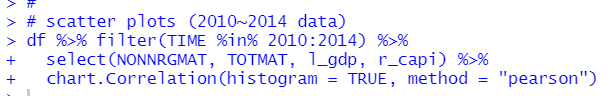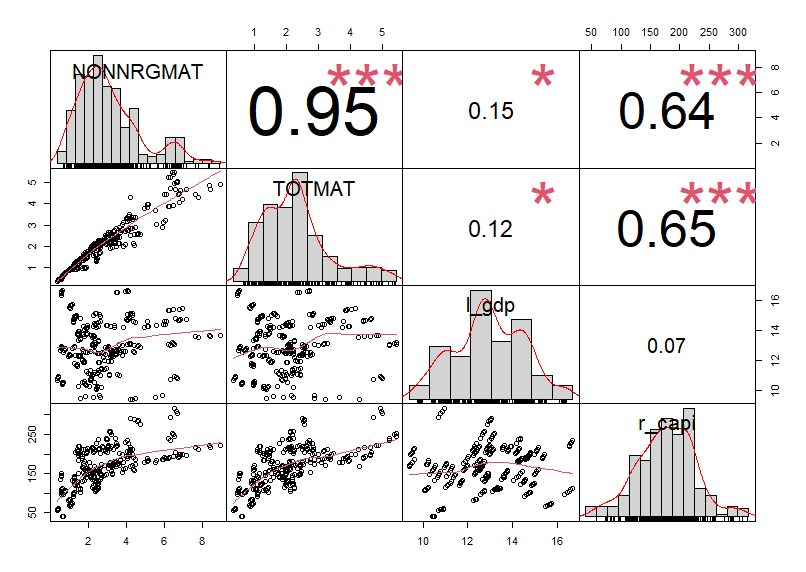NONNRGMAT and TOTMAT has very high correlation.
r_capi and NONNRGMAT, TOTMAT has relatively high correlation.

l_gdp and NONNRGMAT, TOTMAT has weak correlation.

That's it.
Thank you!

The next post is

To see the 1st post,